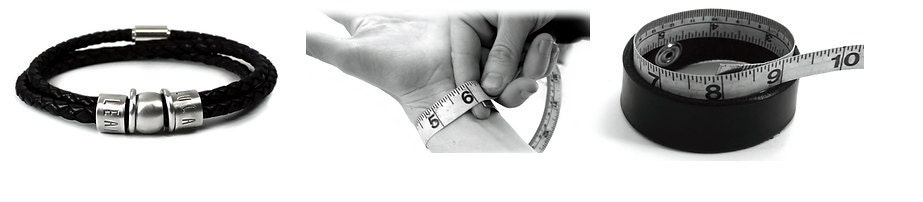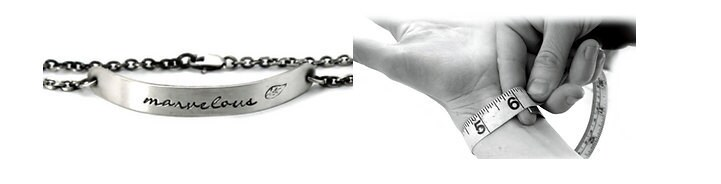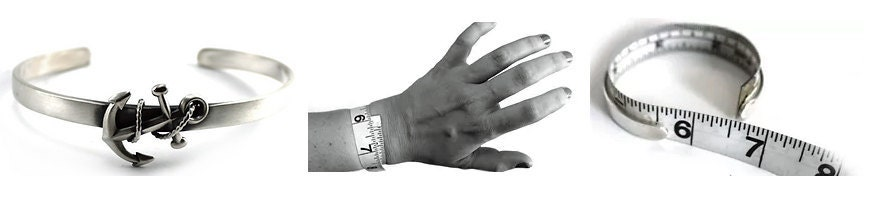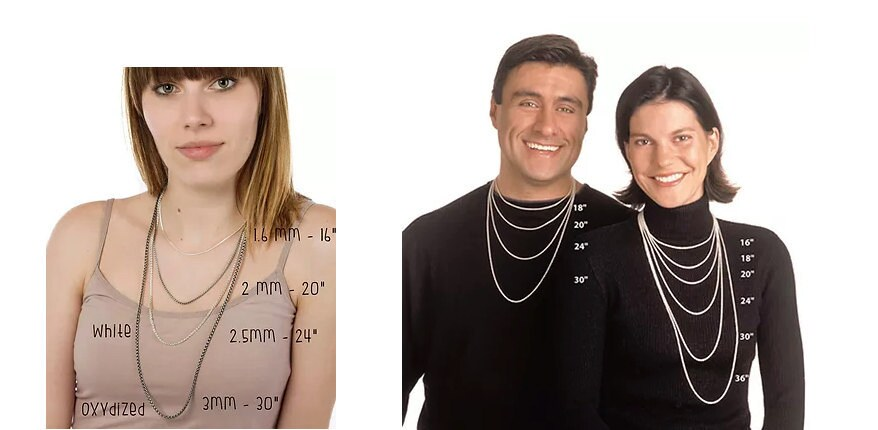## ​

For sizes taken and validated at our Oka boutique:The first size adjustment is free! You have 30 days to return your jewelry and request an adjustment, free of charge.

​Here you will find information for:

Bracelet Size

Open Bangle Size

Bangle Size

Ring Size

Here are some tips to help you know your ring size.​IN JEWELERYThe BEST way to know your exact finger size is to go to a jewelry store and try on a ring about the same width as the one you want to buy.

### Plastic ring sizer to know your ring size

C\$5.00Price

Livraison gratuite View Details

## ​

FROM A RING

If you have a ring of the right size, it is possible to know the size you need using a ruler. The ring should have approximately the same diameter (width of the circle) as the one you wish to purchase. If the ring on which you take the measurement is thin and you want to order a large bangle, add 1/2 size to your size and vice versa.(Example: a size 6 of a narrow ring (3 mm or less) is equivalent to a size 6 1/2 for a ring of 5 mm or more.)

This method is not the best nor the one I recommend the most, but it works relatively well if the process is done well. This is a good technique if you're giving a ring as a gift and it's a surprise.

You will need a sheet, a precise pencil and a millimeter ruler.

1.  Place the ring on a sheet of paper.

2.  Trace the INSIDE edge of the ring with a precise pencil.

3.  Measure the diameter* of the circle drawn OUTSIDE OF THE pencil LINES.

*The diameter (∅) represents the widest segment of the circle passing through the center.

Here are the different sizes with the corresponding inside diameter (∅):

∅Size 4.00 = 14.78 mm

∅Size 4.25 = 15.00 mm

∅Size 4.50 = 15.28 mm

∅Size 4.75 = 15.40 mm

∅ Size 5.00 = 15.72 mm

∅Size 5.25 = 15.80 mm

∅Size 5.50 = 16.16 mm

∅Size 5.75 = 16.30 mm

∅ Size 6.00 = 16.58 mm

∅Size 6.25 = 16.70 mm

∅Size 6.50 = 17.04 mm

∅Size 6.75 = 17.10 mm

∅ Size 7.00 = 17.30 mm

∅Size 7.25 = 17.50 mm

∅Size 7.50 = 17.70 mm

∅Size 7.75 = 17.90 mm

∅ Size 8.00 = 18.23 mm

∅Size 8.25 = 18.40 mm

∅Size 8.50 = 18.59 mm

∅Size 8.75 = 18.75 mm

∅ Size  = 18.90 mm

∅Size 9.25 = 19.20 mm

∅Size 9.50 = 19.44 mm

∅Size 9.75 = 19.60 mm

∅ Size 10.00 = 19.88 mm

∅Size 10.25 = 20.00 mm

∅Size 10.50 = 20.27 mm

∅Size 10.75 = 20.40 mm

∅ Size 11.00 = 20.60 mm

∅Size 11.25 = 20.80 mm

∅Size 11.50 = 21.13 mm

∅Size 11.75 = 21.20 mm

∅ Size 12.00 = 21.45 mm

∅Size 12.25 = 21.60 mm

∅Size 12.50 = 21.80 mm

∅Size 12.75 = 22.00 mm

∅ Size 13.00 = 22.30 mm

∅Size 13.25 = 22.40 mm

∅Size 13.50 = 22.60 mm

∅Size 13.75 = 22.80 mm

∅ Size 14.00 = 23.14 mm

∅Size 14.25 = 23.30 mm

∅Size 14.50 = 23.50 mm

∅Size 14.75 = 23.70 mm

∅ Size 15.00 = 23.88 mm ∅​​​

Wristband size

Here are some tips to help you know your bracelet size.​LEATHER STRAPThe chosen size corresponds precisely to the inner circumference of your bracelet. To know the size you need, measure the circumference of the wrist, leaving about 1 finger of space between the tape and the wrist.To take the measurement from a bracelet, close it and measure the inside circumference. DO NOT MEASURE BRACELET LENGTH; the thickness being variable, the measurement would be distorted. The tape measure can be replaced with a strip of paper or string which can then be measured flat.CHAIN BRACELET

The size chosen corresponds precisely to the length of your bracelet. To know the size you need, measure the circumference of the wrist, leaving about 1 finger of space between the tape and the wrist.To take the measurement from a bracelet of the same type, measure it flat.  ​​RIGID OPEN BRACELET

The size chosen corresponds precisely to the length of your bracelet. To know the size you need, measure the circumference of the wrist WITHOUT ANY SPACE between the tape and the wrist.To take the measurement from a bracelet of the same type, measure inside the bracelet, from one end to the other.BANGLE BRACELET

The chosen size corresponds precisely to the inner circumference of your bracelet. To find the size you need, measure the circumference of your hand at the widest point, pulling the tape tight around the knuckles and thumb. To take the measurement from a bracelet of the same type, measure the inside circumference of the latter.CHAIN SIZE

The chosen size corresponds precisely to the length of your chain. To know the size you need, it is best to measure it on you with a tape or from a chain you already have. Here are some photos of the different lengths, as an example.​​